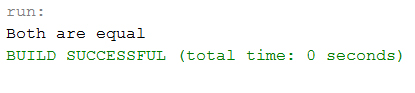# Java else-if Statements

else if statements in Java is like another if condition, it's used in the program when if statement having multiple decisions.

The basic format of else if statement is:

Syntax:
``````if(test_expression)
{
}
else if(test_expression n)
{
}
else
{
}``````

### Example of a Java Program to Demonstrate else If statements

Example:
``````public class Sample {

public static void main(String args[]) {
int a = 30, b = 30;

if (b > a) {
System.out.println("b is greater");
}
else if(a > b){
System.out.println("a is greater");
}
else {
System.out.println("Both are equal");
}
}
}``````
Program Output: